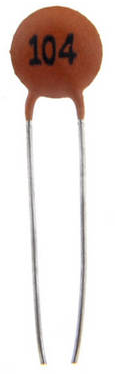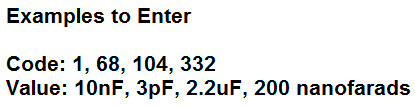﻿ Capacitor Code Calculator ﻿# Capacitor Code CalculatorEnter the Capacitor Code or Value Code ValueThis capacitor code calculator calculates the capacitance value of the capacitor if the code is input or calculates the code of the capacitor if the capacitance value is input.

The capacitor code calculator is used for ceramic capacitors. Ceramic capacitors are the type of capacitors that use this encoding system. Electrolytic capacitors simply have the valiue of the capacitor directly on them. Ceramic capacitors are encoded because they have less surface area on their frame.

So the ceramic capacitor encoding system has 1-3 digits.

If the capacitor only has 1 digit, the capacitance value is that 1 digit in picofarads. So, for example, if you simply see a '6' on the capacitor, then this equals a value of 6 picofarads (pF).

If the capacitor only has 2 digits, the capacitance value are those 2 digits in picofarads. So, for example if you see '68' on the capacitor, then this equals a value of 68 picofarads (pF).

The norm which you will see with ceramic capacitors, though, is that they will have 3 digits.

The first 2 digits are values of the capacitor in picofarads and the third digit is the multiplier factor. Know that the first 2 digits represent the value in picofarads and the 3rd digit is the multiplier factor of the first 2 digits to give the final capacitance value.

The last digit can range in values from 0 to 6. The value cannot exceed 6 for the third digit.

If a capacitor has a third digit of 0, this equals a multiplier factor of 1.

So, for example, if the digits are '680', this equals a capacitance value of 68pF. The first 2 digits are '68' and the multiplier factor is 1, so the value is 68pF (68pF x 1= 68pF).

If a capacitor has a third digit of 1, this equals a multiplier factor of 10.

So, for example, if the digits are '681', this equals a capacitance value of 680pF. The first 2 digits are '68' and the multiplier factor is 10, so the value is 680pF (68pF x 10= 680pF).

If a capacitor has a third digit of 2, this equals a multiplier factor of 100.

So, for example, if the digits are '682', this equals a capacitance value of 6.8nF. The first 2 digits are '68' and the multiplier factor is 100, so the value is 6800pF, or 6.8nF (68pF x 100= 6800pF= 6.8nF).

If a capacitor has a third digit of 3, this equals a multiplier factor of 1000.

So, for example, if the digits are '683', this equals a capacitance value of 68nF. The first 2 digits are '68' and the multiplier factor is 1000, so the value is 68,000pF, or 68nF (68pF x 1000= 68,000pF= 68nF).

If a capacitor has a third digit of 4, this equals a multiplier factor of 10,000.

So, for example, if the digits are '684', this equals a capacitance value of 680nF. The first 2 digits are '68' and the multiplier factor is 10,000, so the value is 680,000pF or 680nF (68pF x 10,000= 680,000pF= 680nF).

If the capacitor's last digit is 5, this equals a multiplier factor of 100,000.

So, for example, if the digits are '685', this equals a capacitance value of 6.8μF. The first 2 digits are '68' and the multiplier factor is 100,000, so the value is 6,800,000pF or 6.8μF (68pF x 100,000= 6,800,000pF= 6.8μF).

If the capacitor's last digit is 6, this equals a multiplier factor of 1,000,000.

So, for example, if the digits are '106', this equals a capacitance value of 10μF. The first 2 digits are '10' and the multiplier factor is 1,000,000, so the value is 10,000,000pF or 10μF (10pF x 1,000,000= 10,000,000pF= 10μF).

Again, for last digit cannot be greater than 6. This is because ceramic capacitor values range from 1pF to 99μF. Rarely do they exceed this. Therefore, you will not see capacitor codes greater than 100μF.

So this is how capacitor code values work.

As stated, this capacitor can convert a code value to the capacitance value or converter the capacitance value to its equivalent code value.

To convert from a code value to a capacitance value, the drop-down box should be set to 'Code'.

To convert from a capacitance value to a code value, the drop-down box should be set to 'Value'.

To enter a value simply, type in the numerical value and then the units. The calculator recognizes the following units: picofarads, pF, nanofarads, nF, microfarads, uF, or μF. So any of these can be entered and the calculator will recognize them.

Related Resources

﻿